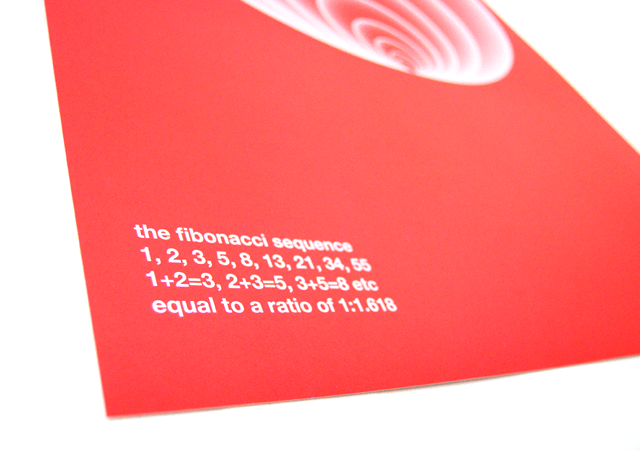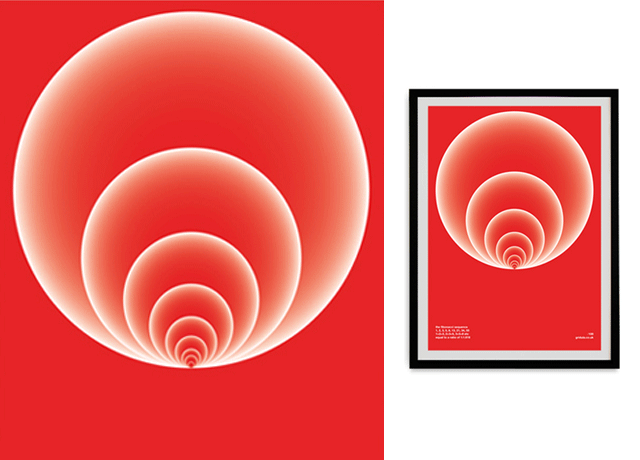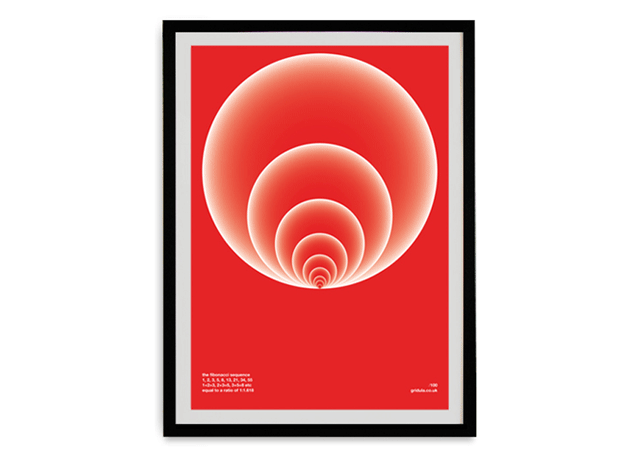•••## Fibonacci Sequence Poster

Visual representation of the Fibonacci Sequence—a mathematical sequence that was introduced by Leonardo of Pisa (AKA Fibonacci) to the West in 1202.

1+2=3, 2+3=5, 3+5=8, 5+8=13, 8+13=21, 13+21=34 is equal to a ratio of 1:1.618, AKA The Golden Ratio which has fascinated Western intellectuals for over 2,400 years.

Unframed A1 Poster printed on 250gsm Greencoat 100% recycled stock in Pantone 032. Limited edition of 100. Each one is hand numbered and shipped in a sturdy 100% recycled tube.

 Postpaid Price UK £28.00 Europe £30.00 World £32.00

### Services Used

• Art Direction
• Design

## Recent Projects

VIEW ALL PROJECTS